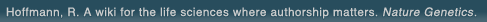# The world's first wiki where authorship really matters (Nature Genetics, 2008). Due credit and reputation for authors. Imagine a global collaborative knowledge base for original thoughts. Search thousands of articles and collaborate with scientists around the globe.

wikigene or wiki gene protein drug chemical gene disease author authorship tracking collaborative publishing evolutionary knowledge reputation system wiki2.0 global collaboration genes proteins drugs chemicals diseases compound
Hoffmann, R. A wiki for the life sciences where authorship matters. Nature Genetics (2008)# Orthogonal array design as a chemometric method for the optimization of analytical procedures. Part 5. Three-level design and its application in microwave dissolution of biological samples.

The theory and methodology of a three-level orthogonal array design as a chemometric method for the optimization of analytical procedures were developed. In the theoretical section, firstly, the matrix of a three-level orthogonal array design is described and orthogonality is proved by a quadratic regression model. Next, the assignment of experiments in a three-level orthogonal array design and the use of the triangular table associated with the corresponding orthogonal array matrix are illustrated, followed by the data analysis strategy, in which significance of the different factor effects is quantitatively evaluated by the analysis of variance (ANOVA) technique and the percentage contribution method. Then, a quadratic regression equation representing the response surface is established to estimate each factor that has a significant influence. Finally, on the basis of the quadratic regression equation established, the derivative algorithm is used to find the optimum value for each variable considered. In the application section, microwave dissolution for the determination of selenium in biological samples by hydride generation atomic absorption spectrometry is employed, as a practical example, to demonstrate the application of the proposed three-level orthogonal array design in analytical chemistry.